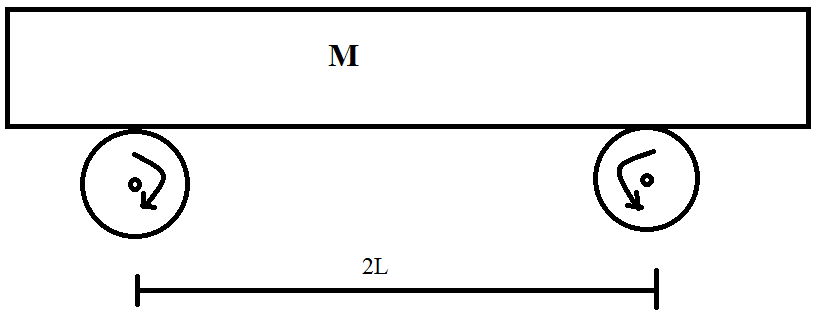A classical mechanics problem by Rishabh Deep SinghTwo wheels which are rotated by some external source with constant angular velocity ($\omega = \pi \text{ units}$) in opposite directions as shown in the figure above. A uniform plank of mass $M = 1\text{ units}$ is placed symmetrically. The friction coefficient between each wheel and the plank is $\mu = 1\text{ units}$. Find the frequency of oscillation, when the plank is slightly displaced along its length ($L=1 \text{ units}$) and relapsed.

Details and Assumptions:

• $g = \pi^2 \text{ units}$.

• Neglect end effect caused by extra length of plank.

×